• +91 9971497814
• info@interviewmaterial.com

# RD Chapter 11- Constructions Ex-11.2 Interview Questions Answers

### Related Subjects

Question 1 : Construct a triangle of sides 4 cm, 5 cm and 6 cm and then a triangle similar to it whose sides are (2/3) of the corresponding sides of it.

Steps of construction :
(i) Draw a line segment BC = 5 cm.
(ii) With centre B and radius 4 cm and with centre C and radius 6 cm, draw arcs intersecting each other at A.
(iii) Join AB and AC. Then ABC is the triangle.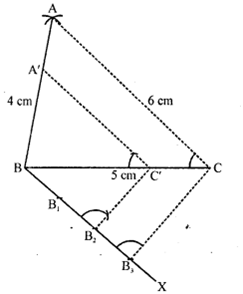(iv) Draw a ray BX making an acute angle with BC and cut off 3 equal parts making BB1 = B1B2= B2B3.
(v) Join B3C.
(vi) Draw B’C’ parallel to B3C and C’A’ parallel to CA then ΔA’BC’ is the required triangle.

Question 2 : Construct a triangle similar to a given ΔABC such that each of its sides is (5/7)th of the corresponding sides of ΔABC. It is given that AB = 5 cm, BC = 7 cm and ∠ABC = 50°.

Steps of construction :
(i) Draw a line segment BC = 7 cm.
(ii) Draw a ray BX making an angle of 50° and cut off BA = 5 cm.
(iii) Join AC. Then ABC is the triangle.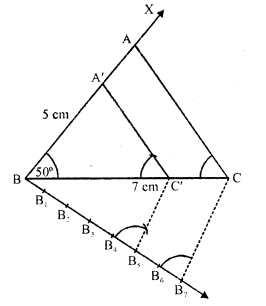(iv) Draw a ray BY making an acute angle with BC and cut off 7 equal parts making BB, =B1B2=B2B3=B3B4=B4Bs=B5B6=B6B7
(v) Join B7 and C
(vi) Draw B5C’ parallel to B7C and C’A’ parallel to CA.
Then ΔA’BC’ is the required triangle.

Question 3 : Construct a triangle similar to a given ∠ABC such that each of its sides is 2/3rd of the corresponding sides of ΔABC. It is given that BC = 6 cm, ∠B = 50° and ∠C = 60°.

Steps of construction :
(i) Draw a line segment BC = 6 cm.
(ii) Draw a ray BX making an angle of 50° and CY making 60° with BC which intersect each other at A. Then ABC is the triangle.
(iii) From B, draw another ray BZ making an acute angle below BC and intersect 3 equal parts making BB1 =B1B2 = B2B2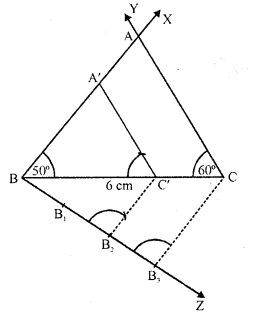(iv) Join B3C.
(v) From B2, draw B2C’ parallel to B3C and C’A’ parallel to CA.
Then ΔA’BC’ is the required triangle.

Question 4 : Draw a ΔABC in which BC = 6 cm, AB = 4 cm and AC = 5 cm. Draw a triangle similar to ΔABC with its sides equal to 3/4th of the corresponding sides of ΔABC.

Steps of construction :
(i) Draw a line segment BC = 6 cm.
(ii) With centre B and radius 4 cm and with centre C and radius 5 cm, draw arcs’intersecting eachother at A.
(iii) Join AB and AC. Then ABC is the triangle,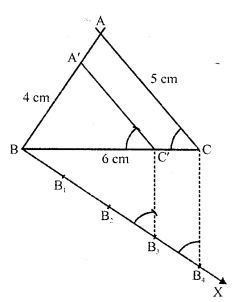(iv) Draw a ray BX making an acute angle with BC and cut off 4 equal parts making BB1=  B1B2 = B2B3 = B3B4.
(v) Join B4 and C.
(vi) From B3C draw C3C’ parallel to B4C and from C’, draw C’A’ parallel to CA.
Then ΔA’BC’ is the required triangle.

Question 5 : Construct a triangle with sides 5 cm, 6 cm and 7 cm and then another triangle whose sides are 7/5 of the corresponding sides of the first triangle.

Steps of construction :
(i) Draw a line segment BC = 5 cm.
(ii) With centre B and radius 6 cm and with centre C and radius 7 cm, draw arcs intersecting eachother at A.
(iii) Join AB and AC. Then ABC is the triangle.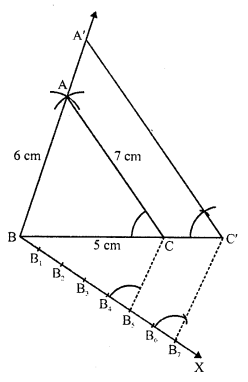(iv) Draw a ray BX making an acute angle with BC and cut off 7 equal parts making BB1 = B1B2 = B2B3 = B3B4 = B4B5 = B5B6 = B6B7.
(v) Join B5 and C.
(vi) From B7, draw B7C’ parallel to B5C and C’A’ parallel CA. Then ΔA’BC’ is the required triangle.

Question 6 : Draw a right triangle ABC in which AC = AB = 4.5 cm and ∠A = 90°. Draw a triangle similar to ΔABC with its sides equal to (54)th ot the corresponding sides of ΔABC.

Steps of construction :
(i) Draw a line segment AB = 4.5 cm.
(ii) At A, draw a ray AX perpendicular to AB and cut off AC = AB = 4.5 cm.
(iii) Join BC. Then ABC is the triangle.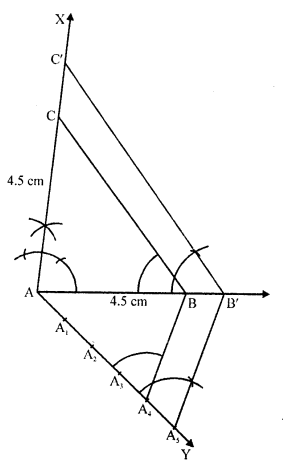(iv) Draw a ray AY making an acute angle with AB and cut off 5 equal parts making AA1 = A1A2 = A2A3 =A3A4 = A4A5
(v) Join A4 and B.
(vi) From 45, draw 45B’ parallel to  A4B and  B’C’ parallel to BC.
Then ΔAB’C’ is the required triangle.

Question 7 : Draw a right triangle in which the sides (other than hypotenuse) are of lengths 5 cm and 4 cm. Then construct another triangle whose sides are 5/3 times the corresponding sides of the given triangle.  (C.B.S.E. 2008)

Steps of construction :
(i) Draw a line segment BC = 5 cm.
(ii) At B, draw perpendicular BX and cut off BA = 4 cm.
(iii )join Ac , then ABC is the triangle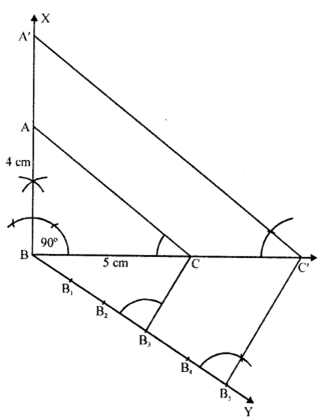(iv) Draw a ray BY making an acute angle with BC, and cut off 5 equal parts making BB1 = B1B2 = B2B3 = B3B4 = B4B5
(v) Join B3 and C.
(vi) From B5, draw B5C’ parallel to B3C and C’A’ parallel to CA.
Then ΔA’BC’ is the required triangle.

Question 8 : Construct an isosceles triangle whose base is 8 cm and altitude 4 cm and then another triangle whose sides are 3/2 times the corresponding sides of the isosceles triangle.

Steps of construction :
(i) Draw a line segment BC = 8 cm and draw its perpendicular bisector DX and cut off DA = 4 cm.
(ii) Join AB and AC. Then ABC is the triangle.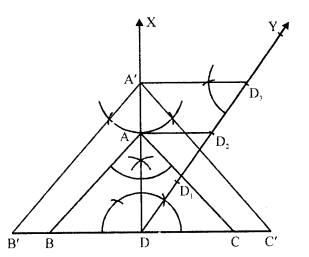(iii) Draw a ray DY making an acute angle with OA and cut off 3 equal parts making DD1 = D1D2 =D2D3 = D3D4
(iv) Join D2
(v) Draw D3A’ parallel to D2A and A’B’ parallel to AB meeting BC at C’ and B’ respectively.
Then ΔB’A’C’ is the required triangle.

Question 9 : Draw a ΔABC with side BC = 6 cm, AB = 5 cm and ∠ABC = 60°. Then construct a trianglewhose sides are (3/4)th of the corresponding sides of the ΔABC.

Steps of construction :
(i) Draw a line segment BC = 6 cm.
(ii) At B, draw a ray BX making an angle of 60° with BC and cut off BA = 5 cm.
(iii) Join AC. Then ABC is the triangle.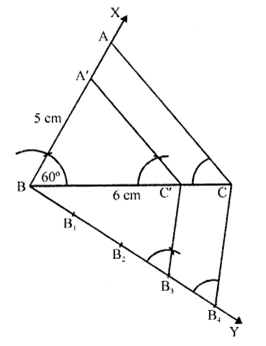(iv) Draw a ray BY making an acute angle with BC and cut off 4 equal parts making BB1= B1B2  B2B3=B3B4.
(v) Join B4 and C.
(vi) From B3, draw B3C’ parallel to B4C and C’A’ parallel to CA.
Then ΔA’BC’ is the required triangle.

Question 10 : Construct a triangle similar to ΔABC in which AB = 4.6 cm, BC = 5.1 cm,∠A = 60° with scale factor 4 : 5.

Steps of construction :
(i) Draw a line segment AB = 4.6 cm.
(ii) At A, draw a ray AX making an angle of 60°.
(iii) With centre B and radius 5.1 cm draw an arc intersecting AX at C.
(iv) Join BC. Then ABC is the triangle.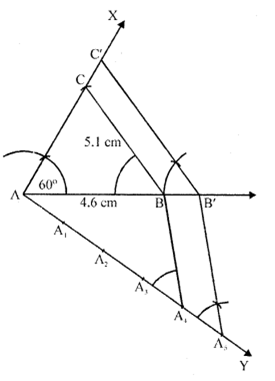(v) From A, draw a ray AX making an acute angle with AB and cut off 5 equal parts making AA1 = A1A2 = A2A3 = A3A4=A4A5.
(vi) Join A4 and B.
(vii) From A5, drawA5B’ parallel to A4B and B’C’ parallel to BC.
Then ΔC’AB’ is the required triangle.

Todays Deals### RD Chapter 11- Constructions Ex-11.2 Contributorskrishan

Name:
Email:

# Latest News# 9000 interview questions in different categories